# How to Split Strings in Pandas: The Beginner's Guide [+ Examples]Updated:

Published:

Good data management relies on good data quality, and consistent formatting is essential to both goals. For example, you are running an ad campaign targeting users based in Nevada. However, half your users abbreviate their state of residence while the others use the full name. If you are not aware of this inconsistency, you could inadvertently segment your list by pulling records that match "NV." If that happens, you are only engaging half your prospects, and business outcomes suffer.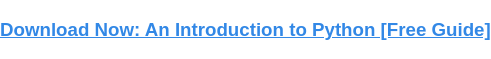Splitting strings of related data into separate cells in columns is a common part of cleansing and formatting data for upload to a data store. This process is necessary not only to make the data easier to query but to ensure compliance with the standards and schemas in highly structured databases such as a data warehouse.

As a Python library specializing in relational (AKA structured) data, pandas provides a built-in function to split strings: the aptly named .split method. This post will review the basic syntax for using .split and then look at three more advanced use cases for splitting strings in your DataFrames.

## Pandas Split String Into Columns

Let's start by breaking down the base syntax for .split.

All tutorials in this post will use a DataFrame of basic user information. You can inspect the data below.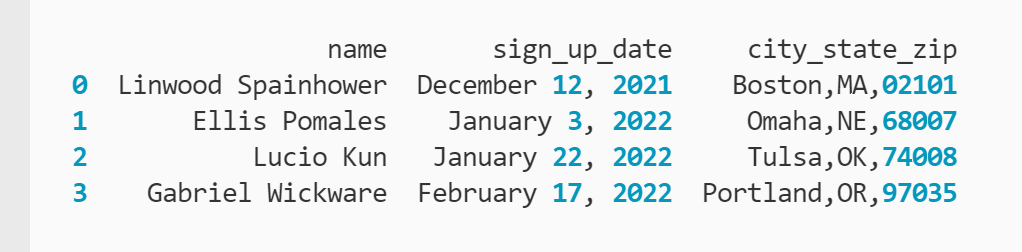All of this data is relevant, but not as efficient for querying in its current format. For example, if you want to find out how many users signed up in 2021, a function can find all records that have values in the "sign_up_date" column that end in 2021.

However, its turnaround time will be slower because it's parsing lots of irrelevant data in each cell. In other words, it must scan the month and day parts of the string before determining if 2021 is included. This may not lead to a noticeable difference in a small DataFrame like this example. But, if your DataFrame has thousands of rows, you may notice a significant delay in turnaround time versus scanning a separate column that only contains year values.

For this tutorial, you want to split the name column into two columns: one for first names and one for last names. To do this, you call the .split() method of the .str property for the "name" column:

``` ```
user_df['name'].str.split()
``````

By default, .split() will split strings where there's whitespace. You can see the output by printing the function call to the terminal: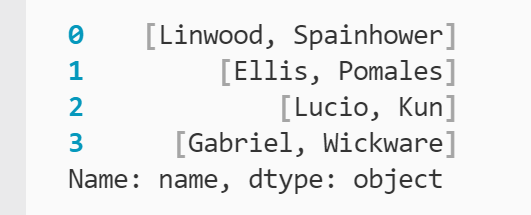You can see .split separated the first and last names as requested. However, the way the divided names are stored as lists in a Series is not very useful. You can break up the lists by using the expand argument setting it to True.

It's also a good idea to be explicit in your code so that others — and your future self — can better understand what's happening. The code below also uses the pat (short for pattern) argument to declare that you are splitting the "name" column values by whitespace (' '):

``` ```
user_df['name'].str.split(pat = ' ', expand = True)
``````

You can confirm .split() performed as expected by examining the result: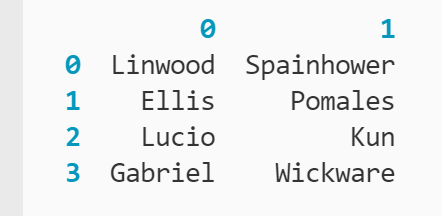Now, you have a DataFrame of two columns for the first and last names of each user. This is closer to the desired result, but you still need to capture this data in the original user_df DataFrame.

To merge the new columns into the user_df DataFrame, you can declare two new columns using the indexing operator ([ ]) and set them equal to the user_names DataFrame:

``` ```
user_names = user_df['name'].str.split(pat = ' ', expand = True)
user_df[['first_name', 'last_name']] = user_names
``````

You can also achieve the same result in one line if you would prefer:

``` ```
user_df[['first_name', 'last_name']] = user_df['name'].str.split(pat = ' ', expand = True)
``````

With either approach, the output is the same: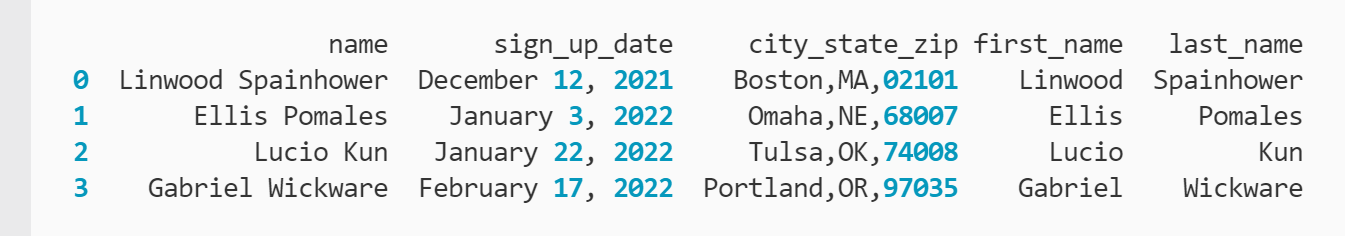At last, the split strings are now captured back in the original DataFrame under the new columns "first_name" and "last_name." However, you may prefer to have these new columns located next to their source column. You can achieve this with the .insert method:

``` ```
user_df.insert(loc = 1, column = 'first_name', value = user_names)
user_df.insert(loc = 2, column = 'last_name', value = user_names)
``````

Here, you are calling .insert() on user_df twice to insert two new columns into the DataFrame. You specify the index of each column with the loc argument, the name for each column, and the list that each column's values should be pulled from. Because user_names contains two different columns, you designate the first (0) or second (1) column with the indexing operator.

.insert places the columns at the specified positions and shifts any existing columns in these locations to the right, ensuring that you don't overwrite any existing data. The result is below.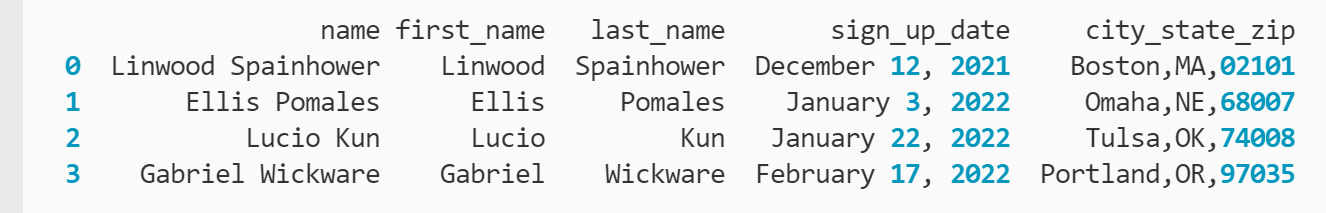Now you have the new "first_name" and "last_name" columns directly behind their source "name" column. If you would like to remove the source column, you can do this with the .drop method:

``` ```
user_df.drop('name', axis = 1, inplace = True)
``````

Here, .drop() searches for the column label "name" based on the axis argument and removes it from the existing user_df DataFrame based on the inplace argument. If you would like a deeper explanation of how .drop works, check out our guide for dropping multiple columns in pandas.

The combined code for this tutorial is below.

``` ```
user_names = user_df['name'].str.split(pat = ' ', expand = True)

user_df.insert(loc = 1, column = 'first_name', value = user_names)
user_df.insert(loc = 2, column = 'last_name', value = user_names)

# OPTIONAL
user_df.drop('name', axis = 1, inplace = True)
``````

Next, let's look at some more complicated examples of the pandas .split method in action.

## Pandas String Split Examples

### 1. Split date strings.

In this scenario, you want to break up the date strings into their composite pieces: month, day, and year. The DataFrame is below for reference.To start breaking up the full date, you return to the .split method:

``` ```
month = user_df['sign_up_date'].str.split(pat = ' ', n = 1, expand = True)
``````

Here, you are calling .split() on the "sign_up_date" column to split the string at the first instance of whitespace. Since there are two spaces in the date string, you use the n argument to specify the number of split operations you want to be performed: 1. Since .split() works left to right, this means it will split the string between month and day: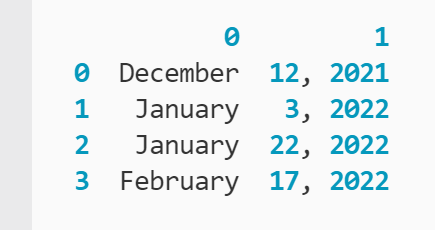However, you still need to split up day and year. Instead of returning to the original DataFrame, you can simplify the workflow by calling .split() on the month DataFrame created by the previous split:

``` ```
day_year = month.str.split(pat = ', ', expand = True)
``````

This time, use the index location (1) to reference the column in month holding the combined day and year strings. By setting pat to ", " instead of only the whitespace, you also ensure that the comma doesn't accompany the day values in the new day_year DataFrame. Since there's only one split location in the day and year string, you no longer need the n argument.

The result of the second split operation is below.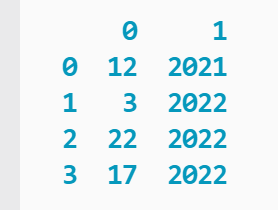You are now ready to populate the original DataFrame with the values from the month and day_year DataFrames using .insert():

``` ```
user_df.insert(loc = 2, column = 'month', value = month)
user_df.insert(loc = 3, column = 'day', value = day_year)
user_df.insert(loc = 4, column = 'year', value = day_year)
``````

Here, the workflow is similar to the previous example with the first and last names. The only notable difference is that you are referencing two different DataFrames for the value argument based on which hold the corresponding pieces of the divided strings.

The DataFrame following the .insert calls is printed below.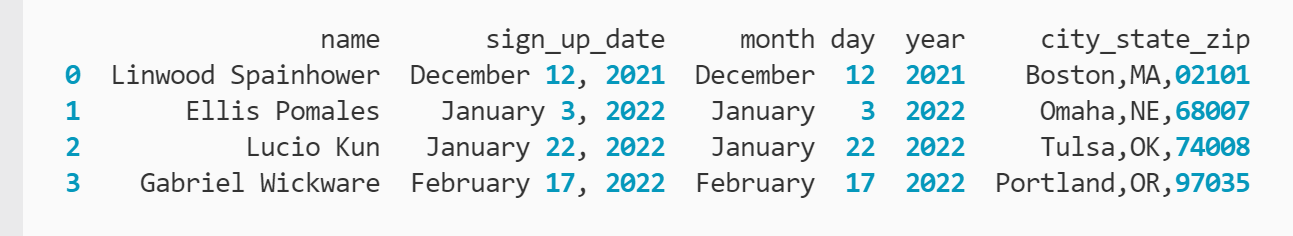The combined code for this example is below.

``` ```
month = user_df['sign_up_date'].str.split(pat = ' ', n = 1, expand = True)
day_year = month.str.split(pat = ', ', expand = True)

user_df.insert(loc = 2, column = 'month', value = month)
user_df.insert(loc = 3, column = 'day', value = day_year)
user_df.insert(loc = 4, column = 'year', value = day_year)
``````

The next section will examine how to split strings based on their index property.

### 2. Split strings by index.

Fortunately, you are not limited to only matching patterns to split strings. You can also use Python's built-in string indexing property to your advantage, particularly when there are no breakpoints (e.g. whitespace, commas, slashes) in the string.

The base DataFrame is below for reference.In this example, you will use string indexing to access the ZIP codes held in the "city_state_zip" column. Because the city names are varying lengths at the front of each string, you can ensure more consistent results by indexing from the back of the string. This means you use negative indexes.

To better understand how negative indexing compares to normal indexing, examine the graphic below: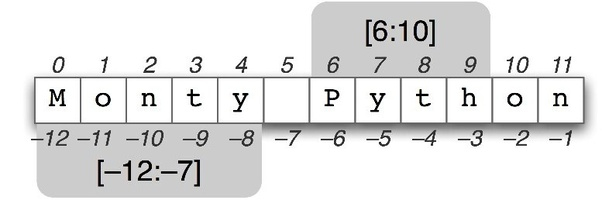Image Source

Based on this graphic, you will want to access the values held in the "city_state_zip" column starting at the -5 through -1 index position, since you know the ZIP codes will always be five characters long. To access the index of each string in the column, you combine the .str property with the indexing operator:

``` ```
zip_codes = user_df['city_state_zip'].str[-5:]
``````

Here, you are declaring a slice with the colon (:) starting at the -5 index position through the -1 index position. Python will assume that you want all characters after -5 if you don't specify an endpoint after the colon. Since slices are exclusive (meaning they include everything up to the end of the range), adding -1 would actually return four-character strings, so the ZIP codes would be incomplete.

With the slice boundaries defined, you are splitting the strings in the "city_state_zip" column at index -5 and saving the result as a Series under the variable zip_codes. The result is printed to the terminal below.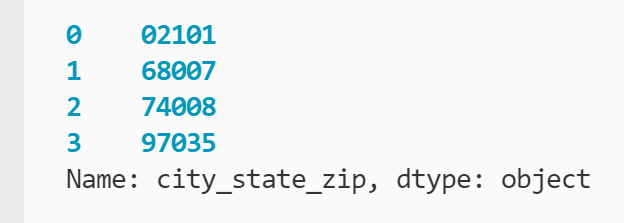Now that you've confirmed the split by index operation worked as expected, you're ready to move this data back into the original DataFrame:

``` ```
user_df['zip'] = zip_codes
``````

This code declares a new column "zip" at the end of the user_df DataFrame and sets it equal to the zip_codes Series. You can confirm it performed as expected by printing the modified DataFrame to the terminal: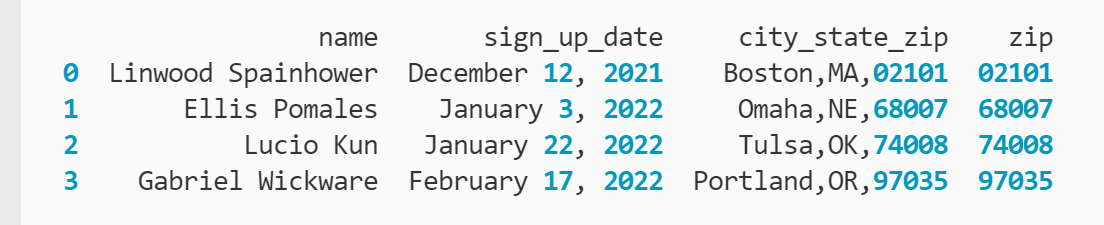The full code for this tutorial is below.

``` ```
zip_codes = user_df['city_state_zip'].str[-5:]

user_df['zip'] = zip_codes
``````

Now, let's take everything that you've learned so far a step further by populating multiple new columns at scale.

### 3. Split strings into multiple columns.

The previous examples have focused more on the .split method and less on the efficient insertion of the data back into the DataFrame. This tutorial will incorporate multiple concepts you've learned in the other tutorials to achieve a consolidated workflow for splitting strings into multiple columns.

For this example, the DataFrame is the same as before, except the signup dates have been reformatted: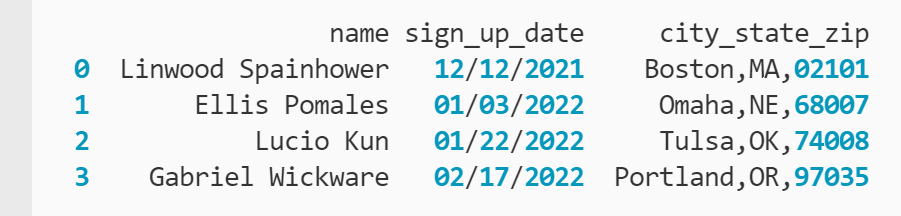The dates are now written integers separated by forward slashes (/). This is a more uniform format than the previous example where months were spelled out and there was no consistent breakpoint between values.

The consistency in the dates' structure also makes it a straightforward process to split them:

``` ```
dates = user_df['sign_up_date'].str.split(pat = '/', expand = True)
``````

.split() is called on the "sign_up_date" column of user_df to split the strings wherever a forward slash (/) occurs. The resulting DataFrame is assigned to the dates variable.

You can confirm .split() performed as expected by printing dates to the terminal: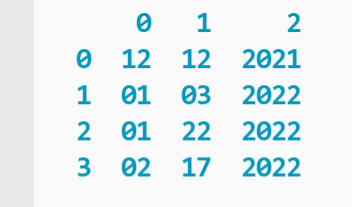Now, let's move beyond appending new columns to the end of the DataFrame or writing multiple insertion statements. Instead, a function will execute the insertion statements for you:

``` ```
def col_loop(df, start_index, name_list, data_df):
i = 0
for name in name_list:
df.insert(
loc = start_index,
column = name,
value = data_df[i]
)
i += 1
start_index += 1
``````

Here, the def keyword declares a new function named col_loop(). col_loop() takes four arguments, which are defined below.

• df: The DataFrame you want to insert the new columns into.
• start_index: The index to start inserting the new columns at. start_index increments to ensure the columns are inserted in the order they are stored in their source DataFrame.
• name_list: A list of column names you want to be assigned to each new column in the DataFrame.
• data_df: The DataFrame generated by .split that the new columns will be pulled from.

After declaring the function, the variable i is declared to serve as a temporary index. A for loop is then declared to cycle through each name in the name_list list. Since name_list will be the same length as the number of new columns to insert, this ensures that the for loop will execute the exact number of times needed.

Inside the for loop, .insert() is called like in previous examples, except the values that you previously defined for each call are now set by the arguments passed in. After .insert() finishes executing, i and start_index increment (increase by one) so that the column order is maintained for the next .insert() call.

So far, you've only defined the function. To use col_loop(), you need to call it:

``` ```
col_loop(user_df, 2, ['month', 'day', 'year'], dates)
``````

You can confirm that col_loop() executed successfully by printing the updated DataFrame: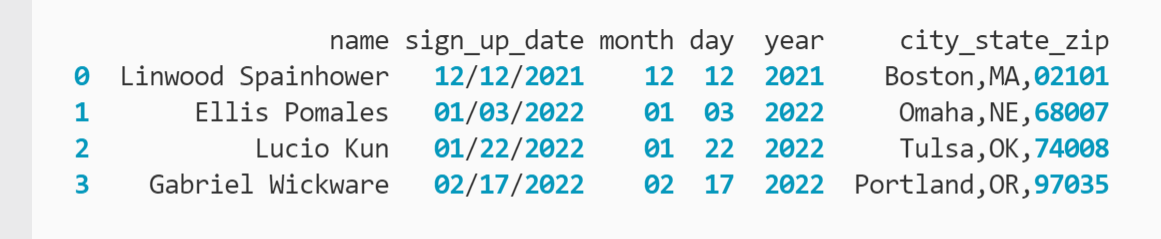The combined code for this example is below.

``` ```
dates = user_df['sign_up_date'].str.split(pat = '/', expand = True)

def col_loop(df, start_index, name_list, data_df):
i = 0
for name in name_list:
df.insert(
loc = start_index,
column = name,
value = data_df[i]
)
i += 1
start_index += 1

col_loop(user_df, 2, ['month', 'day', 'year'], dates)
``````

Excluding the function definition, you've now achieved what took five lines of code in the first example of splitting date strings and condensed it down to two lines.

The added complexity of the function definition and call may not seem worth it to save three lines of code. But what if you needed to insert more than three columns? col_loop() allows you to scale the insertion operation much easier and avoids the greater risk of human error that comes with pasting multiple .insert calls with different arguments. Plus, you can export col_loop() for use in other files so you don't need to define it each time you use it.

## Split strings in Pandas to improve querying and analysis.

Splitting strings is a crucial process for cleansing your data before analysis or preparing it for upload to a data store. Breaking up related data into its components leads to more efficient queries of the data set. In addition, databases may have strict schemas that require data to be formatted in specific ways. Python provides the .split method to address this need within pandas DataFrames and Series to ensure your analysis and data storage are as efficient as possible.Topics: What Is Python?

## Related Articles

We're committed to your privacy. HubSpot uses the information you provide to us to contact you about our relevant content, products, and services. You may unsubscribe from these communications at any time. For more information, check out our Privacy Policy.

A guide for marketers, developers, and data analysts.